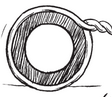Q

# Through a rectangular field of length 90 m and breadth 60 m, two roads are constructed which are parallel to the sides and cut each other at right angles through the centre of the fields. (ii) the cost of constructing the roads

Q. 7.     Through a rectangular field of length and breadth, two roads are constructed which are parallel to the sides and cut each other at right angles through the centre of the fields. If the width of each road is .find

(ii) the cost of constructing the roads at the rate of  .Views
RIt is given that the width of each road is  and the length of rectangular park is  and breadth is

Now, We know that area of rectangle is =

Area of the total park is

-(i)

Area of road parallel to the width of the park ( ABCD ) is

-(ii)

Area of road parallel to the length of park ( PQRS )  is

-(iii)

The common area of both the roads ( KMLN ) is

-(iv)

Now, the cost of constructing the roads at the rate of   is

Therefore, the cost of constructing the roads at the rate of   is

Exams
Articles
Questions